Here's one more thing I want to point out...  This takes us back to when we graphed lines in this form: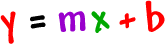When we let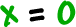, we get the y-intercept (where the graph crosses the y-axis).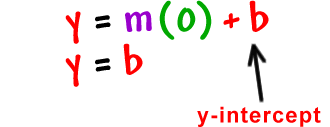We can do the same thing on these!

Check out the first guy we did:Let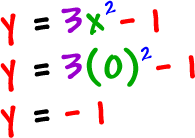Here's the second one we did:Let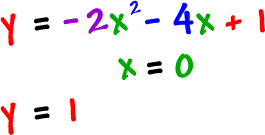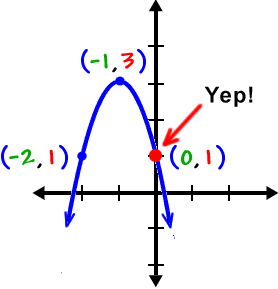Once you get the idea, you can get the y-intercept just by glancing!